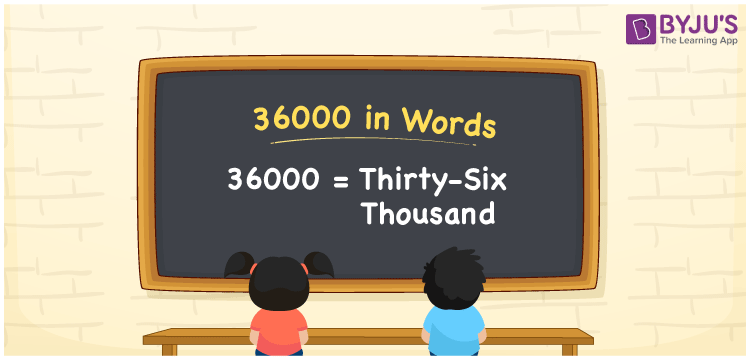# 36000 in Words

36000 in words can be written as Thirty-six thousand. The article on 36000 provides students with a clear idea of counting numbers. For instance, if you borrow Rs. 36000 from your friend, then you can say that “I borrowed Thirty-six thousand rupees from my friend”. This article will provide you with a clear idea of the number 36000 into words in an interesting way. However, English alphabets are used to write numbers in words. So, 36000 can be read as “Thirty-six Thousand” in English.

 36000 in words Thirty-six Thousand Thirty-six Thousand in Numbers 36000

## 36000 in English Words## How to Write 36000 in Words?

Learn how to write 36000 in words from the place value chart provided below. There are five digits in the number 36000. The place value chart indicating the place value for all the five digits is as follows.

 Ten thousands Thousands Hundreds Tens Ones 3 6 0 0 0

The expanded form of 36000 is:

3 x Ten thousand + 6 × Thousand + 0 × Hundred + 0 × Ten + 0 × One

= 3 × 10000 + 6 × 1000  + 0 × 100 + 0 × 10 + 0 × 1

= 30000 + 6000

= 36000

= Thirty-six Thousand

Hence, 36000 in words is written as Thirty-six Thousand.

36000 is a natural number that precedes 36001 and succeeds 35999.

36000 in words – Thirty-six Thousand

Is 36000 an odd number? – No

Is 36000 an even number? – Yes

Is 36000 a perfect square number? – No

Is 36000 a perfect cube number? – No

Is 36000 a prime number? – No

Is 36000 a composite number? – Yes

## Frequently Asked Questions on 36000 in Words

Q1

### How do you write 36000 in words?

In English, 36000 can be written as “Thirty-six thousand”.
Q2

### Is 36000 an even number?

Yes, 36000 is an even number as it is divisible by 2.
Q3

### How do you write Thirty-six thousand in numbers?

Thirty-six thousand in numbers is 36000.